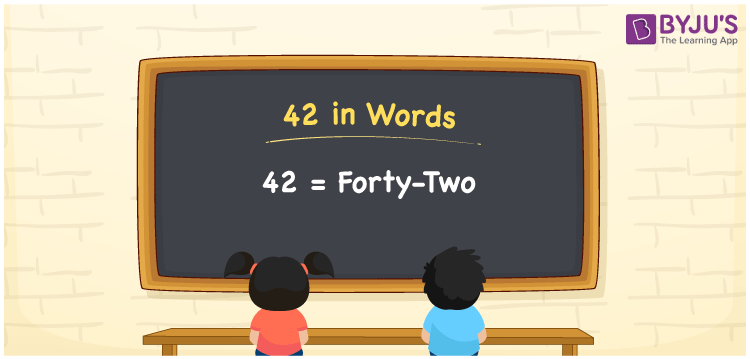# 42 in Words

42 in words is written as “Forty-two”. 42 is a two-digit number that has two place values, ones and tens. It represents a count, quantity or value. To represent 42 in words, we should know how to read and write it in English since it is the most commonly used language. Also, learning to spell numbers in words is necessary to utilise in real-life situations. For example, Arsh saved Forty-two Rupees out of shopping today. Learn how to write 42 in words in this article.

 42 in words Forty-two Forty-two in Numbers 42

## 42 in English Words

We generally write numbers in words using the English alphabet. Thus, we can read 42 in English as “Forty-two”.## How to Write 42 in Words?

To convert 42 in words, we need to check the place value of its digits. Since 42 is a two-digit number, thus, place value of 4 is tens and 2 is ones. The order of place values of digits in a number is in accordance with the Indian numbering system, such as:

• Ones
• Tens
• Hundreds
• Thousands
• Ten-thousands
• Hundred-thousands or Lakhs

This can be done as follows.

 Tens Ones 4 2

This can be written as:

4 × Ten + 2 × One

= 4 × 10 + 2 × 1

= 40 + 2

= 42

= Forty-two

Thus, 42 in words is written as Forty-two.

42 is a natural number that precedes 43 and succeeds 41.

42 in words – Forty-two

Is 42 an odd number? – No

Is 42 an even number? – Yes

Is 42 a perfect square number? – No

Is 42 a perfect cube number? – No

Is 42 a prime number? – No

Is 42 a composite number? – Yes

## Frequently Asked Questions on 42 in Words

Q1

### How do you write 42 in English?

We can write 42 in English words as “Forty-two”.
Q2

### What is 342 in English?

342 is written as Three hundred and forty-two in English.
Q3

### Is 42 an even number or an odd number?

42 is an even number since it is completely divisible by 2.